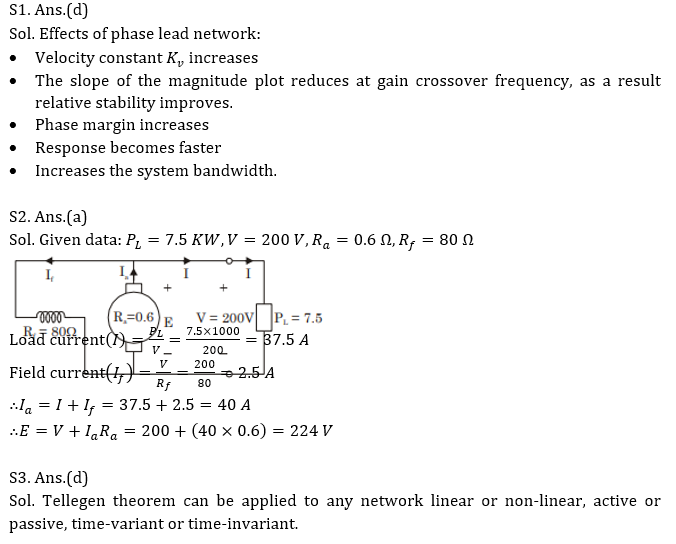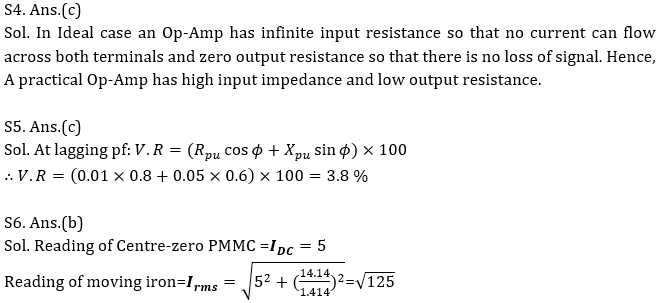Engineering Jobs   »   UPRVUNL JE EE QUIZ

# DFCCIL’21 EE: Daily Practices Quiz 30-July-2021

Each question carries 1 mark.
Negative marking: 1/4 mark
Total Questions: 06
Time: 08 min.

Q1. Which one of the following statements regarding an effect of phase lead network is
NOT correct?
(a) The velocity constant is usually increased
(b) The slope of the magnitude curve is reduced at the gain crossover frequency, as a result relative stability improves
(c) Phase margin increased
(d) The bandwidth decreased

Q2. A DC shunt generator supplies a load of 7.5 kW at 200 V. If the armature resistance is 0.6 Ω and field resistance is 80 Ω, the induced emf will be
(a) 224 V
(b) 218 V
(c) 212 V
(d) 204 V

Q3. Which of the following theorems can be applied to any network linear or non-linear, active or passive, time-variant or time-invariant?
(a) Norton theorem
(b) Thevenin theorem
(c) Superposition theorem
(d) Tellegen theorem

Q4. An operational amplifier possesses
(a) very large input resistance and very large output resistance
(b) very small input resistance and very small output resistance
(c) very large input resistance and very small output resistance
(d) very small input resistance and very large output resistance

Q5. If the pu impedance of a single-phase transformer is 0.01+j0.05, then its regulation at 0.8 lagging will be:
(a) 2.2 %
(b) -2.2 %
(c) 3.8 %
(d) -3.8 %

Q6. A current i=5+14.14 sin⁡(ωt+45^0) is passed through a center-zero PMMC and a moving-iron instrument respectively, the respective readings are:
(a) -5 and 15
(b) 5 and √125
(c) -5 and 19.14
(d) 5 and 10

SOLUTIONSSharing is caring!